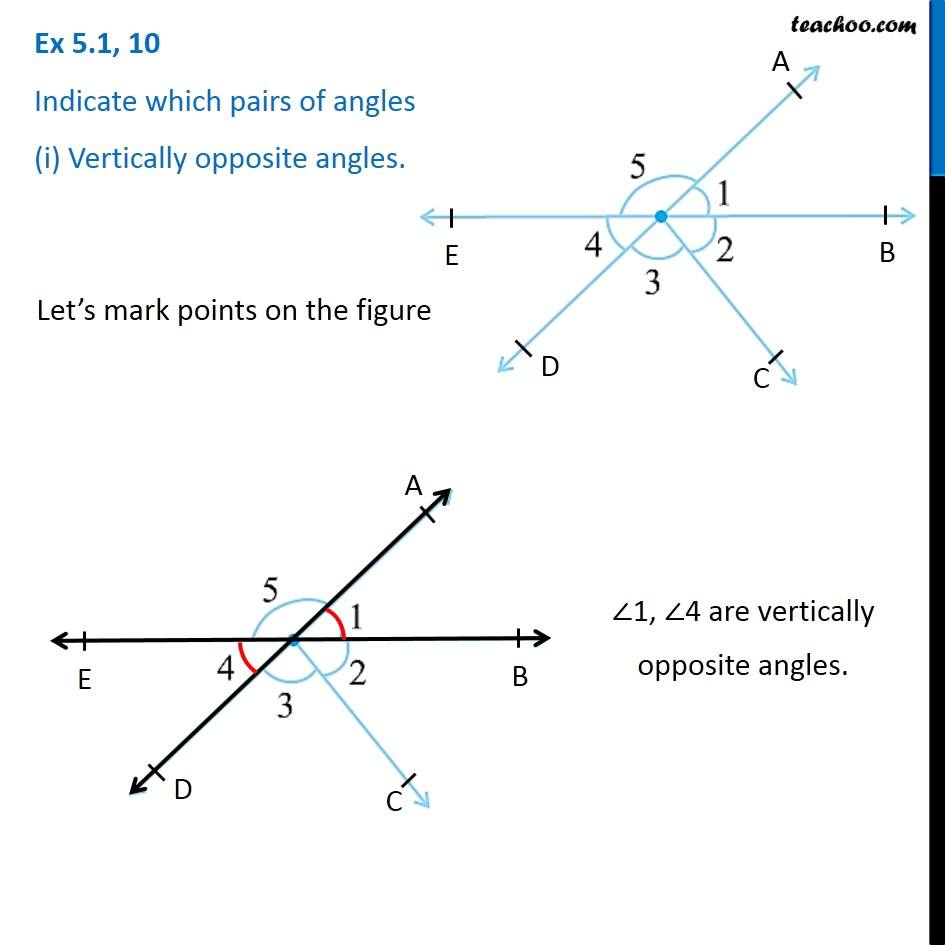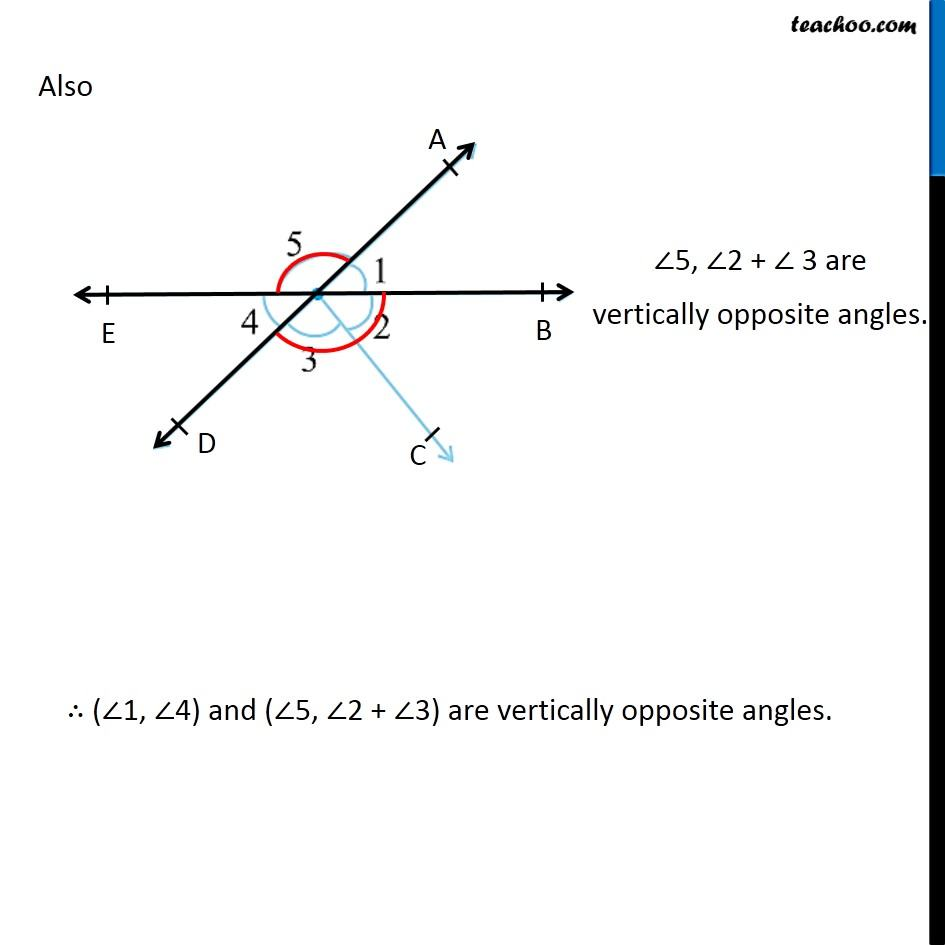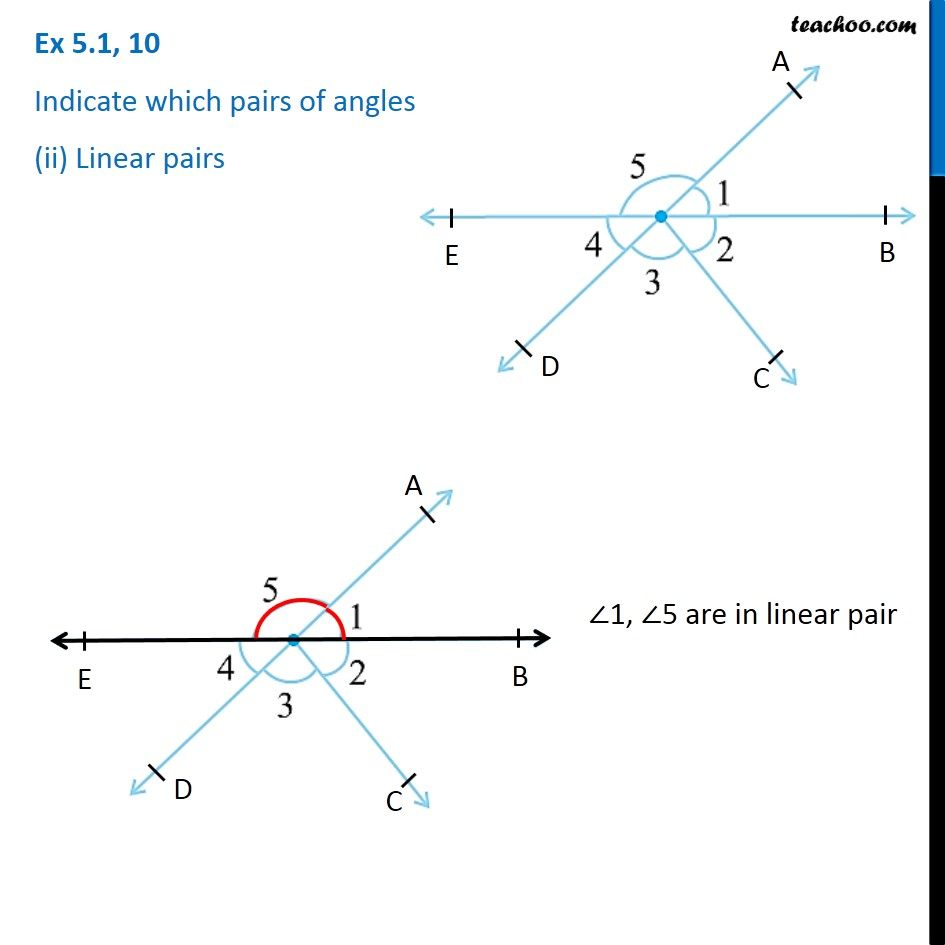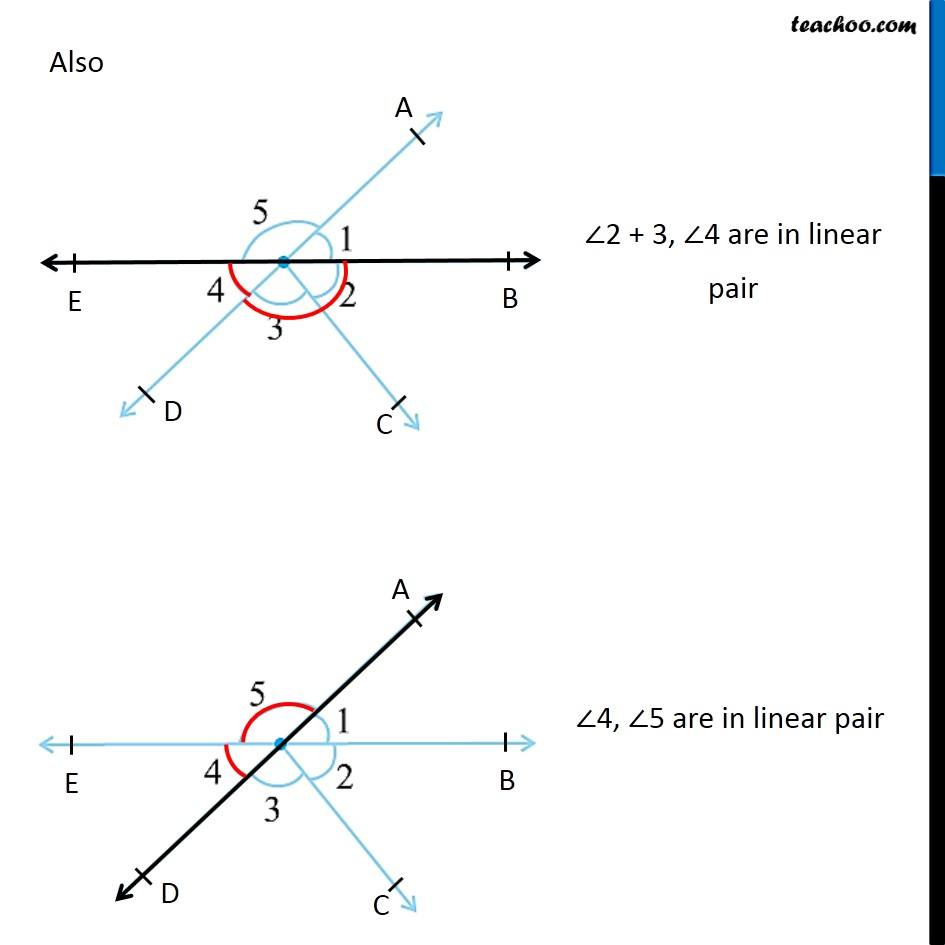1. Chapter 5 Class 7 Lines and Angles
2. Concept wise
3. Adjacent Angles, Linear Pair of angles, Vertically Opposite angles

Transcript

Ex 5.1, 10 Indicate which pairs of angles are: (i) Vertically opposite angles.Let’s mark points on the figure ∠1, ∠4 are vertically opposite angles. Also ∠5, ∠2 + ∠ 3 are vertically opposite angles. ∴ (∠1, ∠4) and (∠5, ∠2 + ∠3) are vertically opposite angles. Ex 5.1, 10 Indicate which pairs of angles are: (ii) Linear pairs∠1, ∠5 are in linear pair Also ∠2 + 3, ∠4 are in linear pair ∠4, ∠5 are in linear pair

Adjacent Angles, Linear Pair of angles, Vertically Opposite angles